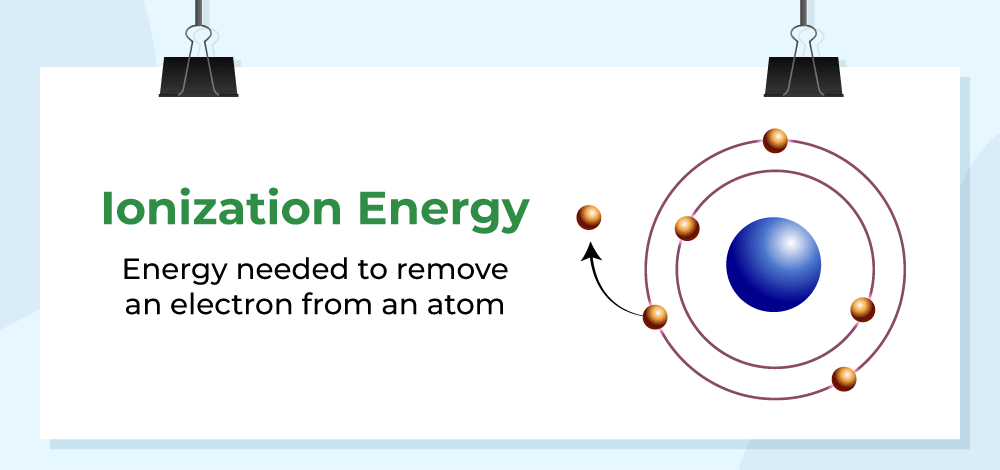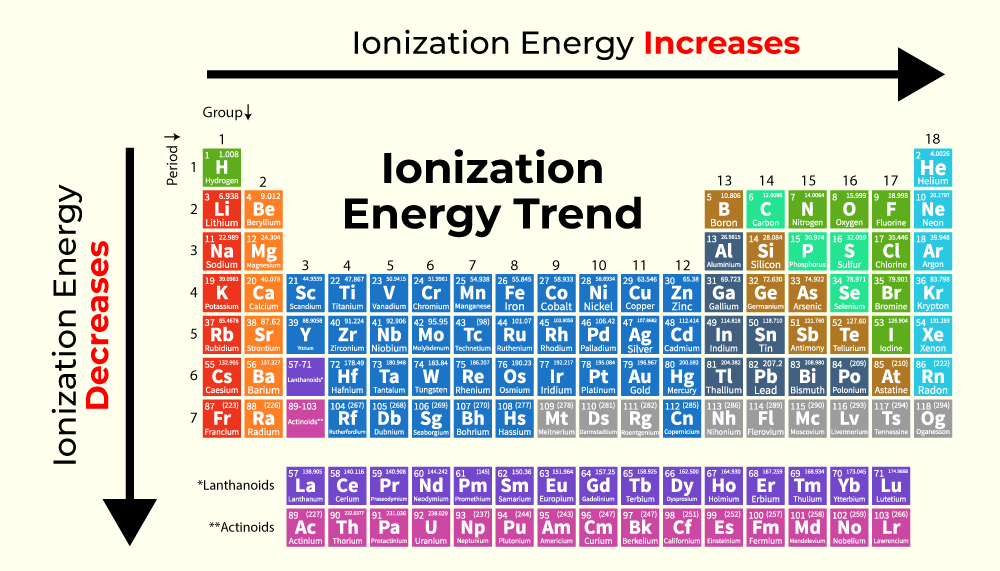GFG App
Open AppBrowser
Continue

Ionization energy can be defined as the minimum amount of energy required to remove the valence electron i.e. the most loosely bound electron from an isolated gaseous atom or ion. It is important to note the term isolated gaseous atom as if the atom is not isolated, then the energy required may be more due to the interatomic forces.

## What is Ionization Energy?

Ionization Energy is defined as the difficulty in removing the electron from an atom or ion.

X + Δ ⟶  X+ + e

Ionization is an endothermic process as the energy needs to be supplied in order to remove the electron. The ionization energy increases as the removal of electrons takes place due to the fact that the atom now gains a +ve charge and the electrons are held more tightly.  Thus it is difficult to remove an electron from a cation.

Ionization energy or ionization potential is measured in electronvolts (eV) or KJ/mol. eV is a preferred unit in physics while kJ/mol is a preferred unit in chemistry as chemistry generally deals with 1 mole of an atom.

## Factors Affecting Ionization Energy

Factors that affect the Ionization Energy of any atom and molecule are:

• If an atom or ion is positively charged then the electrons are more tightly held by the nucleus, so the ionization energy is high.
• If the number of shells is less i.e. the electrons are near to the nucleus then the IE is high and vice versa.
• It is difficult to remove the inner electrons as compared to the outer electrons due to this reason.
• The IE is less when there is more than 1 electron in a shell due to the interelectronic repulsions.
• An atom with lower IE acts as a good reducing agent while those with high IE act as a good oxidizing agent.

## Ionization Energy using Bohr’s Atomic Model

According to Bohr’s model of the atom, electrons can exist only in fixed paths called orbits. The electrons revolve around the nucleus in these fixed orbits due to the attraction of the nucleus. Each orbit has its own fixed energy. The electron in a particular orbit is said to possess the energy of the same orbit. If an electron gains energy equal to the energy difference between two orbits then it can jump to the higher orbit whose energy it possesses. This is called the excitation of the electron. If an electron gains enough energy, then it can overcome the attractive force of the nucleus and finally come out of the atom. Such an atom is said to be ionized.

The image given below shows the Ionization energy of the electron.## Ionization Energy Formula

Thus the ionization energy is the energy difference between the energy of the electron in the initial orbit and the energy of the electron outside the atom (infinite orbit in this case).

According to Bohr, the energy of an electron in the nth orbit is given by:where,
m is mass of one electron,
e is charge on one electron,
h is Planck’s constant,
Z is atomic number,
n is the orbit from which electron is removed

This formula can be rewritten as,

En ​=−R × n2 / Z2

En = −13.6 × n2 / Z2 eV / atom

This is the energy of the atom in the nth orbit. Now we said that the ionization energy is the energy difference between the energy of the electron in the initial orbit and the energy of the electron in the infinite orbit. Thus we can consider the initial orbit as n1​ = n and the final orbit as n2 = ∞.

IE = En2​​ − En1​​ = ΔEAsThis formula is known as the ionization energy formula. Note that the IE is the same as the energy of the electron in the nth orbit but positive in sign. This is due to the fact that the energy is supplied to remove the electron and thus it has a positive sign.

## How to Determine the Ionization Energy of an Element?

The Ionization Energy of an Element of an element is calculated using the steps given below,

Step 1: First write the atomic number of the atom for which the Ionization energy has to be found.

Step 2: Determine ‘n’ the value of the orbit for which ionization energy is to be found.

Step 3: Use the formula for finding the Ionization Energy. En = −13.6 × n2 / Z2 eV / atom

Step 4: Substitute all the values in the above formula and simplify to get Ionization Energy in electron volts.

## Successive Ionization Energies

An atom can have any number of ionization energies depending on the number of electrons it has.

### First Ionization Energy

Energy required to remove the first outermost electron from a neutral atom is known as the first IE. The first IE is equal to the energy of the electron in that particular orbit.

### Second Ionization Energy

The energy required to remove the second electron from an atom with +1 charge is called second IE and so on. The second Ionization energy is always higher than the first IE as it is difficult to remove the electron from a positively charged ion.

X + ΔH1 ⟶ X+ + e

where ΔH1 = First IE

X+ + ΔH2 ⟶ X+2 + e

where ΔH2​ = Second IE

The IE keeps on increasing as the charge on the atom increases. Thus the third IE is higher than the second and the second IE is higher than the first IE.

ΔH1 < ΔH2 < ΔH3 …… < ΔHn

## Ionization Energy Trends in Periodic Table

Ionization Energy follows a specific trend while moving from Left to Right and Top to Bottom in the periodic table.

The image given below shows the trend of variation of Ionization Energy while moving left to right and top to bottom in the periodic table.### Left to Right in a Period

As we move from left to right in a period in the periodic table the atomic radius decreases. It is due to the reason that the elements start gaining near noble gas configuration as we move across the period. Due to this interelectronic repulsion is reduced and the atomic radius decreases. Hence the electrons are more tightly held by the nucleus. Hence a higher amount of energy is required to remove the electrons. Thus IE increases as we move across a period.

### Top to Bottom in a Group

As we move from top to bottom in a group, new shells are added up and the electrons start to occupy higher orbitals and shells. Thus the atomic radius also increases. The electrons are now far away from the nucleus. So lesser amount of energy is required to remove the electron. Thus IE decreases as moves down a group.

## Solved Example on Ionization Energy

Example 1: Calculate the ionization energy of the Hydrogen atom if the electron is in the second orbit.

Solution:

For hydrogen given, Z = 1, n = 2

Thus,

ΔE = 13.6 × n2 / Z2

= 13.6 × 1/4

= 3.4 eV

Example 2: Find the IE required to remove the outermost electron from the Sodium atom.

Solution:

For Sodium, Z=11 and n=3 for outermost electron

Thus,

ΔE = 13.6 × n2 / Z2

= 13.6 × 121/9

= 182.844 eV

Example 3: Calculate the wavelength of radiation required to ionize the Na atom if it is known that the ionization energy of Na is 496 kJ/mol.

Solution:

Given,

ΔE = 496 kJ/mol,

λ = ?

We know that E = hc / λ

496 = (6.6 × 10−34 × 3 × 108) / λ

λ = 3.99 ×10−28 ms−1

Example 4: The first, second, and third ionization energies of Aluminium are 578, 1817, and 2745 kJ/mol. Calculate the ionization energy required to convert Al ion to Al3+.

Given,

ΔH1 = 578, ΔH2 = 1817, ΔH3 = 2745

Al + ΔE ⟶  Al3+

ΔE = ΔH1 + ΔH2 + ΔH3

ΔE = 578+1817+2745

= 5140 kJ/mol

## FAQs on Ionization Energy

### Q1: What is Ionization Energy?

Ionization energy can be defined as the minimum amount of energy required to remove the valence electron i.e. the most loosely bound electron from an isolated gaseous atom or ion.

### Q2: What factors govern Ionization Energy?

Factors governing ionization energy are,

• If an atom or ion is positively charged then the electrons are more tightly held by the nucleus, so the ionization energy is high.
• If the number of shells are less i.e. the electrons are near to the nucleus then the IE is high and vice versa.
• The IE is less when there are more than 1 electrons in a shell due to the inter electronic repulsions.

### Q3: Why does the ionization energy increase with the increase in charge?

As the charge on the atom increases, the inter electronic repulsions become less. Thus the electrons are more closer to the nucleus and held more tightly. Thus it becomes difficult to remove the electron and hence the ionization energy increases with the increase in charge of the atom.

### Q4: Which has the highest ionization energy between nitrogen and oxygen?

Nitrogen has the highest ionization energy between nitrogen and oxygen as nitrogen has half-filled electronic configuration so it requires more energy to extract electrons from the Nitrogen’s orbit.